GeeksforGeeks App
Open AppBrowser
Continue

# How to Show Gridlines on Matplotlib Plots?

In this article, we will see how we can add gridlines to a matplotlib graph and various configurable parameters that can be used in Python.

Example:

Create a matplotlib plot using sample data.

## Python

 `# Importing the library``import` `matplotlib.pyplot as plt`` ` `# Define X and Y data points``X ``=` `[``12``, ``34``, ``23``, ``45``, ``67``, ``89``]``Y ``=` `[``1``, ``3``, ``67``, ``78``, ``7``, ``5``]`` ` `# Plot the graph using matplotlib``plt.plot(X, Y)`` ` `# Function to view the plot``plt.show()`

Output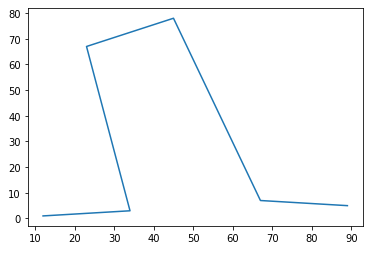## grid()

The grid() method is used to define a grid in a matplotlib graph. The syntax is given by –

Syntax:

matplotlib.pyplot.grid(b=None, which=’major’, axis=’both’, **kwargs)

Parameters:

• b: bool or None, optional: Whether to show the grid lines. If any kwargs are supplied, it is assumed you want the grid on and b will be set to True. If b is None and there are no kwargs, this toggles the visibility of the lines.
• which: {‘major’, ‘minor’, ‘both’}, optional: The grid lines to apply the changes on.
• axis: {‘both’, ‘x’, ‘y’}, optional: The axis to apply the changes on.
• **kwargs: Line2D properties: Define the line properties of the grid

Example 1: Default Gridlines

## Python

 `# Importing the library``import` `matplotlib.pyplot as plt`` ` `# Define X and Y data points``X ``=` `[``12``, ``34``, ``23``, ``45``, ``67``, ``89``]``Y ``=` `[``1``, ``3``, ``67``, ``78``, ``7``, ``5``]`` ` `# Plot the graph using matplotlib``plt.plot(X, Y)`` ` `# Add gridlines to the plot``plt.grid(b``=``True``)``# `plt.grid()` also works`` ` `# Function to view the plot``plt.show()`

Output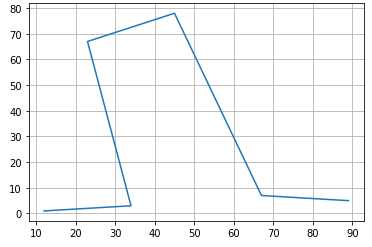Example 2: Apply Gridlines to  x- axis

In the cases, where we would want to see only the vertical or horizontal gridlines, we can make use of the `axis` parameter. To view only vertical gridlines –

Syntax:

`plt.grid(b=True, axis='x')`

## Python3

 `# Importing the library``import` `matplotlib.pyplot as plt`` ` `# Define X and Y data points``X ``=` `[``12``, ``34``, ``23``, ``45``, ``67``, ``89``]``Y ``=` `[``1``, ``3``, ``67``, ``78``, ``7``, ``5``]`` ` `# Plot the graph using matplotlib``plt.plot(X, Y)`` ` `# Add gridlines to the plot``plt.grid(b``=``True``, axis``=``'x'``)``# `plt.grid()` also works`` ` `# Function to view the plot``plt.show()`

Output: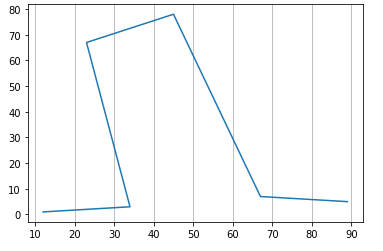Example 3: Apply Gridlines to y-axis

In the cases, where we would want to see only the vertical or horizontal gridlines, we can make use of the `axis` parameter. To view only horizontal gridlines –

Syntax:

` plt.grid(b=True, axis='y')`

## Python3

 `# Importing the library``import` `matplotlib.pyplot as plt`` ` `# Define X and Y data points``X ``=` `[``12``, ``34``, ``23``, ``45``, ``67``, ``89``]``Y ``=` `[``1``, ``3``, ``67``, ``78``, ``7``, ``5``]`` ` `# Plot the graph using matplotlib``plt.plot(X, Y)`` ` `# Add gridlines to the plot``plt.grid(b``=``True``, axis``=``'y'``)``# `plt.grid()` also works`` ` `# Function to view the plot``plt.show()`

Output: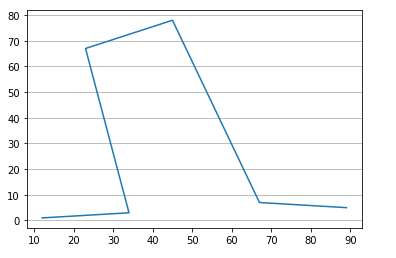Example 4: Provide a linestyle and linewidth

We have seen the gridlines being a solid lines. We can change this to view a different line-style like dashes using an argument `linestyle` or `ls`. Similarly, we can change the thickness of the gridlines using the argument `linewidth`. These arguments are provided as part of the keyword arguments and can be viewed in the document link.

To provide a line style –

Syntax:

`plt.grid(linestyle='--')`

## Python3

 `# Importing the library``import` `matplotlib.pyplot as plt`` ` `# Define X and Y data points``X ``=` `[``12``, ``34``, ``23``, ``45``, ``67``, ``89``]``Y ``=` `[``1``, ``3``, ``67``, ``78``, ``7``, ``5``]`` ` `# Plot the graph using matplotlib``plt.plot(X, Y)`` ` `# Add gridlines to the plot``plt.grid(linestyle``=``'--'``)``# `plt.grid()` also works`` ` `# Function to view the plot``plt.show()`

Output: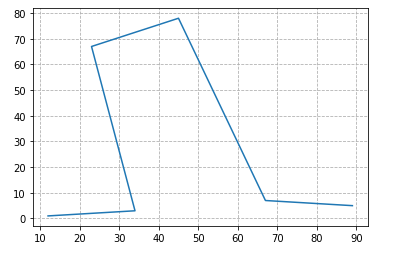.

Example 5: Change color of the Gridlines

The keyword argument `color` can be used to define a different color to the plot.

Syntax:

`plt.grid(linestyle, color)`

## Python3

 `# Importing the library``import` `matplotlib.pyplot as plt`` ` `# Define X and Y data points``X ``=` `[``12``, ``34``, ``23``, ``45``, ``67``, ``89``]``Y ``=` `[``1``, ``3``, ``67``, ``78``, ``7``, ``5``]`` ` `# Plot the graph using matplotlib``plt.plot(X, Y)`` ` `# Add gridlines to the plot``plt.grid(linestyle``=``'--'``, color``=``'pink'``)``# `plt.grid()` also works`` ` `# Function to view the plot``plt.show()`

Output: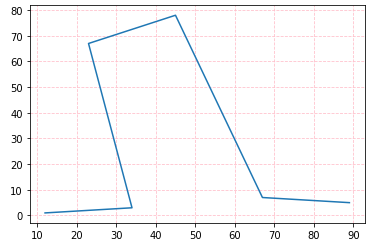My Personal Notes arrow_drop_up